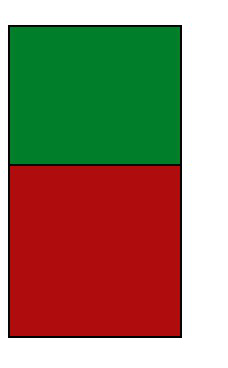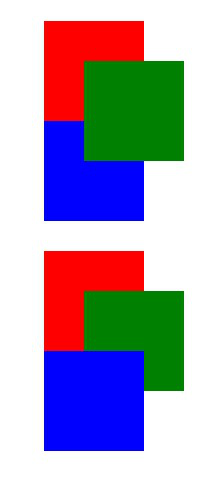Open in App
Not now

# Difference between auto, 0, and no z-index in CSS

• Difficulty Level : Expert
• Last Updated : 31 Oct, 2021

When we have to order elements on the  z-axis, we use the z-index property. in CSS, the z-index property only works on elements with a position value other than static. In this article, we will learn about the z-index property and its values, auto, number, initial and inherit. We will also see the difference between all the values.

Syntax:

`z-index: auto | integer | initial | inherit;`

Properties: This property has the following values as mentioned above and described  below.

• auto: It sets the stack order equal to to its parents. This is the default value.
• integer: It sets the stack order of the element. We can also use negative numbers.
• initial: It sets this property to its default value.
• inherit: It inherits this property from its parent element.

Note: The z-index property does not work on static position elements.

The following examples will help to understand the differences between the values of the property.

Example 1: In this example we will use the z-index property on on element.

## HTML

 ` ` `<``html` `lang``=``"en"``> ` ` `  `<``head``> ` `    ``<``style``> ` `        ``.box { ` `            ``width: 170px; ` `            ``height: 170px; ` `            ``border: 2px solid black; ` `        ``} ` ` `  `        ``#box-1 { ` `            ``top: 69px; ` `            ``position: relative; ` `            ``background-color: rgb(0, 126, 42); ` `            ``z-index: auto; ` `        ``} ` ` `  `        ``#box-2 { ` `            ``top: 34px; ` `            ``position: relative; ` `            ``background-color: rgb(175, 13, 13); ` `        ``} ` `    `` ` ` ` ` `  `<``body``> ` `    ``<``div` `class``=``"box"` `id``=``"box-1"``> ` `    ``<``div` `class``=``"box"` `id``=``"box-2"``> ` ` ` ` `  ``

Output:Example 2: In this example, we will use the z-index property on both the elements.

## HTML

 ` ` `<``html` `lang``=``"en"``> ` ` `  `<``head``> ` `    ``<``style``> ` `        ``.box { ` `            ``width: 170px; ` `            ``height: 170px; ` `            ``border: 2px solid black; ` `        ``} ` ` `  `        ``#box-1 { ` `            ``top: 69px; ` `            ``position: relative; ` `            ``background-color: rgb(0, 126, 42); ` `            ``z-index: auto; ` `        ``} ` ` `  `        ``#box-2 { ` `            ``top: 34px; ` `            ``position: relative; ` `            ``background-color: rgb(175, 13, 13); ` `            ``z-index: auto; ` `        ``} ` `    `` ` ` ` ` `  `<``body``> ` `    ``<``div` `class``=``"box"` `id``=``"box-1"``> ` `    ``<``div` `class``=``"box"` `id``=``"box-2"``> ` ` ` ` `  ``

Output: We can clearly see that there is no difference between z-index auto and no z-index.Example 3: When we specify the z-index as 0, it creates a stacking context.

## HTML

 ` ` `<``html` `lang``=``"en"``> ` ` `  `<``head``> ` `    ``<``style``> ` `        ``.z { ` `            ``position: relative; ` `            ``width: 100px; ` `            ``height: 100px; ` `            ``top: 40px; ` `            ``left: 40px; ` `        ``} ` ` `  `        ``.red { ` `            ``background: red; ` `        ``} ` ` `  `        ``.green { ` `            ``background: green; ` `        ``} ` ` `  `        ``.blue { ` `            ``background: blue; ` `        ``} ` ` `  `        ``#example2 { ` `            ``margin-top: 30px; ` `        ``} ` `    `` ` ` ` ` `  `<``body``> ` `    ``<``div` `id``=``"example1"``> ` `        ``<``div` `class``=``"z red"``> ` `            ``<``div` `class``=``"z green"`  `                 ``style``=``"z-index: 1"``> ` `        `` ` `        ``<``div` `class``=``"z blue"``> ` `    `` ` ` `  `    ``<``div` `id``=``"example2"``> ` `        ``<``div` `class``=``"z red"`  `             ``style``=``"z-index: 0"``> ` `            ``<``div` `class``=``"z green"`  `                 ``style``=``"z-index: 1"``> ` `              `` ` `        `` ` `        ``<``div` `class``=``"z blue"``> ` `    `` ` ` `  ` ` ` `  ``

Output:Explanation of the examples: In the first example, we can see that green is positioned above blue and red, this is due to the reason that the color green has z-index 1. Hence a stacking context is created.

But in the second example, green is positioned above red but below blue due to the reason that red has z-index 0. Hence a stacking context is created at the same level as blue.

My Personal Notes arrow_drop_up
Related Articles